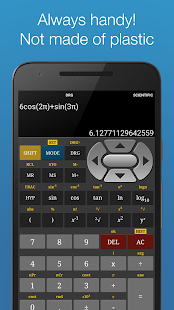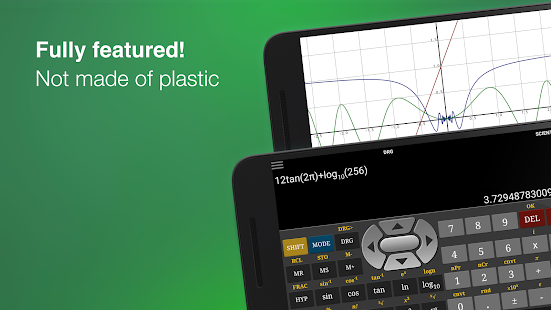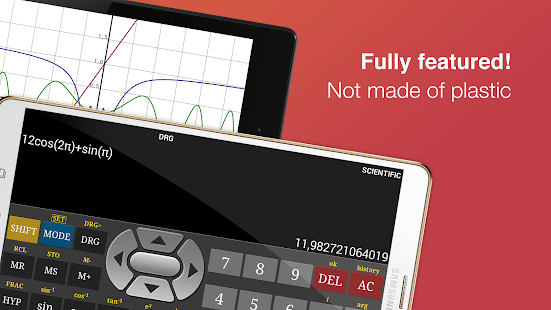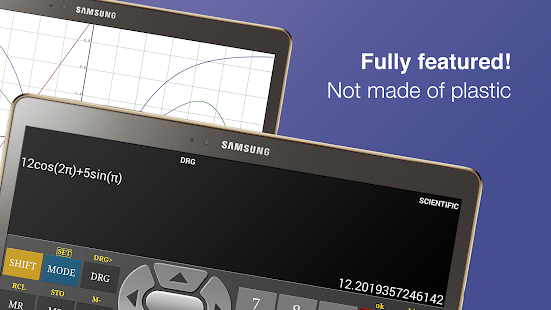# Scientific Calculator Pro v6.8.0 [Latest]

Report this app

## DescriptionThis is a fully featured scientific and graphing calculator which supports graph, matrix, complex numbers, equation solver and unit converter. For everyday calculations, the calculator also supports a basic mode.

Features :

• Large input/output display
• Dedicated Graph Plotter
• Complex number
• Full Matrix Support up to order of 9×9
• Simultaneous linear equation solver up to 9 variables
• Polynomial equation solver – gives both real and complex solution
• Landscape Mode
• Calculation History
• All Trigonometric operations
• Binary, Decimal, Octal, Hexadecimal operation
• Bitwise operations
• Ten memory locations
• Results history feature
• Constants Table
• Built-in help (accessible via options menu

Screenshots

••••••••